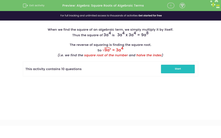# Find the Square Roots of Algebraic Terms

In this worksheet, students will simplify the square roots of algebraic terms.Key stage:  KS 3

Curriculum topic:   Algebra

Curriculum subtopic:   Use and Interpret Algebraic Notation

Difficulty level:#### Worksheet Overview

When we find the square of an algebraic term, we simply multiply it by itself.

Thus the square of  3a4 is   3a4 x 3a4 = 9a8

Or,   (3a4)2 = 32 x a4x2 = 9a8

The reverse of squaring is finding the square root..

So,  √(9a8) = √9 x a8÷2 = 3a4

The steps we need to remember are:

1) √ the number

2) Halve the power.

Let's try sme questions now.

### What is EdPlace?

We're your National Curriculum aligned online education content provider helping each child succeed in English, maths and science from year 1 to GCSE. With an EdPlace account you’ll be able to track and measure progress, helping each child achieve their best. We build confidence and attainment by personalising each child’s learning at a level that suits them.

Get started